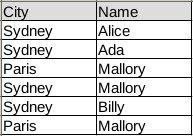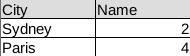Pandas groupby

DataFrames can be summarized using the groupby method. In this article we’ll give you an example of how to use the groupby method.

Related course:
Data Analysis with Python Pandas

Pandas groupby
Start by importing pandas, numpy and creating a data frame. Our data frame contains simple tabular data:In code the same table is:

We can then summarize the data using the groupby method:

This will count the frequency of each city and return a new data frame:The total code being: